You'll be needing to solve some trig equations at the very end of some Calculus problems...  So, let's review!

 Solve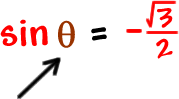Remember that this is asking for thewhere the sine is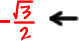That's long and negative!

ALWAYS draw a quick unit circle!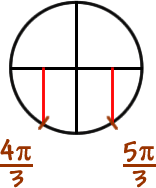Usually, you'll just need to find the solutions on...  which is one lap around the unit circle.

 So, foror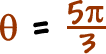If you want ALL the solutions, remember that you add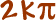to get all the laps aroundor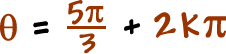Solve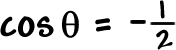onSolveon*hint: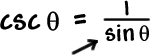find this!